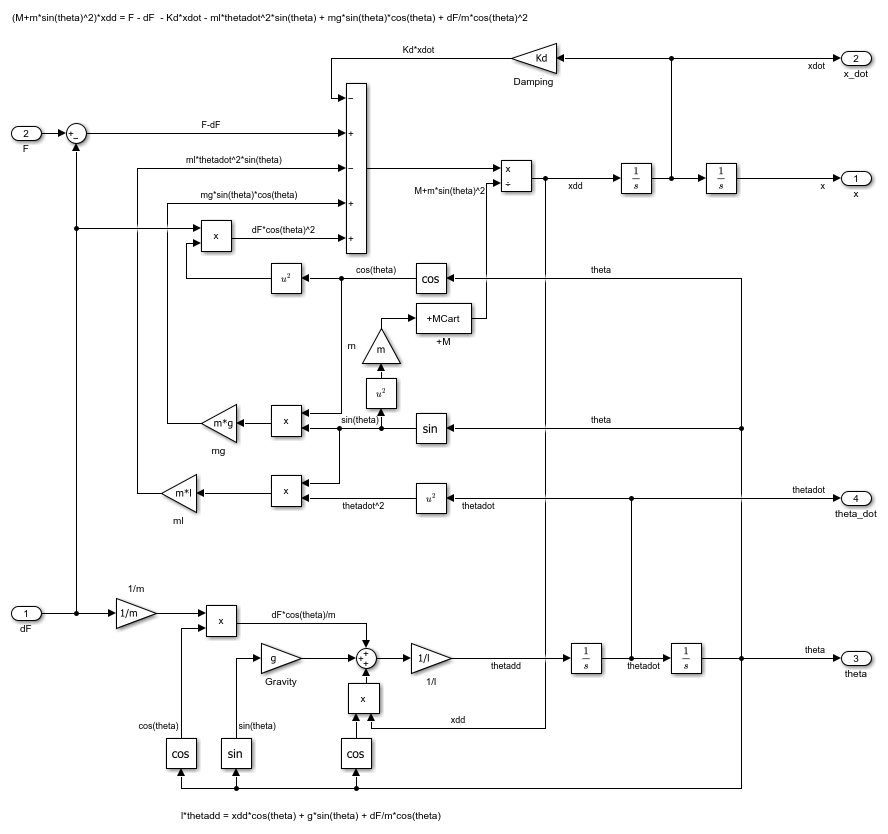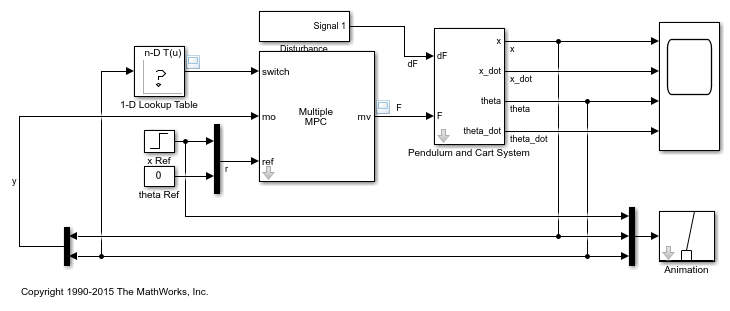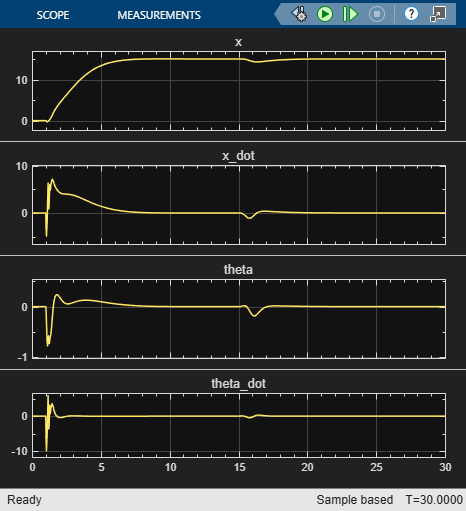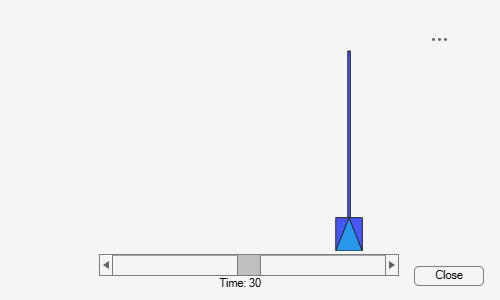Documentation

## Gain-Scheduled MPC Control of an Inverted Pendulum on a Cart

This example uses a gain-scheduled model predictive controller to control an inverted pendulum on a cart.

### Product Requirement

This example requires Simulink® Control Design™ software to define the MPC structure by linearizing a nonlinear Simulink model.

```if ~mpcchecktoolboxinstalled('slcontrol') disp('Simulink Control Design is required to run this example.') return end ```

Add example file folder to MATLAB® path.

```addpath(fullfile(matlabroot,'examples','mpc','main')); ```

### Pendulum/Cart Assembly

The plant for this example is the following cart/pendulum assembly, where x is the cart position and theta is the pendulum angle.This system is controlled by exerting a variable force F on the cart. The controller needs to keep the pendulum upright while moving the cart to a new position or when the pendulum is nudged forward by an impulse disturbance dF applied at the upper end of the inverted pendulum.

This plant is modeled in Simulink with commonly used blocks.

```mdlPlant = 'mpc_pendcartPlant'; load_system(mdlPlant) open_system([mdlPlant '/Pendulum and Cart System'],'force') ```### Control Objectives

Assume the following initial conditions for the cart/pendulum assembly:

• The cart is stationary at x = `0`.

• The inverted pendulum is stationary at the upright position theta = `0`.

The control objectives are:

• Cart can be moved to a new position between `-15` and `15` with a step setpoint change.

• When tracking such a setpoint change, the rise time should be less than 4 seconds (for performance) and the overshoot should be less than `5` percent (for robustness).

• When an impulse disturbance of magnitude of `2` is applied to the pendulum, the cart should return to its original position with a maximum displacement of `1`. The pendulum should also return to the upright position with a peak angle displacement of `15` degrees (`0.26` radian).

The upright position is an unstable equilibrium for the inverted pendulum, which makes the control task more challenging.

### The Choice of Gain-Scheduled MPC

In Control of an Inverted Pendulum on a Cart, a single MPC controller is able to move the cart to a new position between -10 and 10. However, if you increase the step setpoint change to 15, the pendulum fails to recover its upright position during the transition.

To reach the longer distance within the same rise time, the controller applies more force to the cart at the beginning. As a result, the pendulum is displaced from its upright position by a larger angle such as `60` degrees. At such angles, the plant dynamics differ significantly from the LTI predictive model obtained at theta = `0`. As a result, errors in the prediction of plant behavior exceed what the built-in MPC robustness can handle, and the controller fails to perform properly.

A simple workaround to avoid the pendulum falling is to restrict pendulum displacement by adding soft output constraints to theta and reducing the ECR weight on constraint softening.

```mpcobj.OV(2).Min = -pi/2; mpcobj.OV(2).Max = pi/2; mpcobj.Weights.ECR = 100; ```

However, with these new controller settings it is no longer possible to reach the longer distance within the required rise time. In other words, controller performance is sacrificed to avoid violation of soft output constraints.

To move the cart to a new position between -15 and 15 while maintaining the same rise time, the controller needs to have more accurate models at different angles so that the controller can use them for better prediction. Gain-scheduled MPC allows you to solve a nonlinear control problem by designing multiple MPC controllers at different operating points and switching between them at run time.

### Control Structure

For this example, use a single MPC controller with:

• One manipulated variable: Variable force F.

• Two measured outputs: Cart position x and pendulum angle theta.

• One unmeasured disturbance: Impulse disturbance dF.

```mdlMPC = 'mpc_pendcartGSMPC'; open_system(mdlMPC) ```Although cart velocity x_dot and pendulum angular velocity theta_dot are available from the plant model, to make the design case more realistic, they are excluded as MPC measurements.

While the cart position setpoint varies (step input), the pendulum angle setpoint is constant (`0` = upright position).

### Linear Plant Model

Since the MPC controller requires a linear time-invariant (LTI) plant model for prediction, linearize the Simulink plant model at three different operating points.

Specify linearization input and output points

```io(1) = linio([mdlPlant '/dF'],1,'openinput'); io(2) = linio([mdlPlant '/F'],1,'openinput'); io(3) = linio([mdlPlant '/Pendulum and Cart System'],1,'openoutput'); io(4) = linio([mdlPlant '/Pendulum and Cart System'],3,'openoutput'); ```

Create specifications for the following three operating points, where both cart and pendulum are stationary:

• Pendulum is at 80 degrees, pointing right (theta = `-4*pi/9`)

• Pendulum is upright (theta = `0`)

• Pendulum is at 80 degrees, pointing left (theta = `4*pi/9`)

```angles = [-4*pi/9 0 4*pi/9]; for ct=1:length(angles) ```

Create operating point specification.

``` opspec(ct) = operspec(mdlPlant); ```

The first state is cart position x.

``` opspec(ct).States(1).Known = true; opspec(ct).States(1).x = 0; ```

The second state is cart velocity x_dot (not at steady state).

``` opspec(ct).States(2).SteadyState = false; ```

The third state is pendulum angle theta.

``` opspec(ct).States(3).Known = true; opspec(ct).States(3).x = angles(ct); ```

The fourth state is angular velocity theta_dot (not at steady state).

``` opspec(ct).States(4).SteadyState = false; ```
```end ```

Compute operating points using these specifications.

```options = findopOptions('DisplayReport',false); [op,opresult] = findop(mdlPlant,opspec,options); ```

Obtain the linear plant model at the specified operating points.

```plants = linearize(mdlPlant,op,io); ```
```bdclose(mdlPlant) ```

### Multiple MPC Designs

At each operating point, design an MPC controller with the corresponding linear plant model.

```status = mpcverbosity('off'); for ct=1:length(angles) ```

Get a single plant model.

``` plant = plants(:,:,ct); plant.InputName = {'dF'; 'F'}; plant.OutputName = {'x'; 'theta'}; ```

The plant has two inputs, dF and F, and two outputs, x and theta. In this example, dF is specified as an unmeasured disturbance used by the MPC controller for prediction. Set the plant signal types.

``` plant = setmpcsignals(plant,'ud',1,'mv',2); ```

To control an unstable plant, the controller sample time cannot be too large (poor disturbance rejection) or too small (excessive computation load). Similarly, the prediction horizon cannot be too long (the plant unstable mode would dominate) or too short (constraint violations would be unforeseen). Use the following parameters for this example:

``` Ts = 0.01; PredictionHorizon = 50; ControlHorizon = 5; mpcobj = mpc(plant,Ts,PredictionHorizon,ControlHorizon); ```

Specify nominal input and output values based on the operating point.

``` mpcobj.Model.Nominal.Y = [0;opresult(ct).States(3).x]; mpcobj.Model.Nominal.X = [0;0;opresult(ct).States(3).x;0]; mpcobj.Model.Nominal.DX = [0;opresult(ct).States(2).dx;0;opresult(ct).States(4).dx]; ```

There is a limitation on how much force we can apply to the cart, which is specified as hard constraints on manipulated variable F.

``` mpcobj.MV.Min = -200; mpcobj.MV.Max = 200; ```

It is good practice to scale plant inputs and outputs before designing weights. In this case, since the range of the manipulated variable is greater than the range of the plant outputs by two orders of magnitude, scale the MV input by 100.

``` mpcobj.MV.ScaleFactor = 100; ```

To improve controller robustness, increase the weight on the MV rate of change from `0.1` to `1`.

``` mpcobj.Weights.MVRate = 1; ```

To achieve balanced performance, adjust the weights on the plant outputs. The first weight is associated with cart position x and the second weight is associated with angle theta.

``` mpcobj.Weights.OV = [1.2 1]; ```

To achieve more aggressive disturbance rejection, increase the state estimator gain by multiplying the default disturbance model gains by a factor of `10`.

Update the input disturbance model.

``` disturbance_model = getindist(mpcobj); setindist(mpcobj,'model',disturbance_model*10); ```

Update the output disturbance model.

``` disturbance_model = getoutdist(mpcobj); setoutdist(mpcobj,'model',disturbance_model*10); ```

Save the MPC controller to the MATLAB workspace.

``` assignin('base',['mpc' num2str(ct)],mpcobj); ```
```end mpcverbosity(status); ```

### Closed-Loop Simulation

Validate the MPC design with a closed-loop simulation in Simulink.

```open_system([mdlMPC '/Scope']) sim(mdlMPC) ```
```-->Converting model to discrete time. -->The "Model.Noise" property of the "mpc" object is empty. Assuming white noise on each measured output channel. -->Converting model to discrete time. -->The "Model.Noise" property of the "mpc" object is empty. Assuming white noise on each measured output channel. -->Converting model to discrete time. -->The "Model.Noise" property of the "mpc" object is empty. Assuming white noise on each measured output channel. ```In the nonlinear simulation, all the control objectives are successfully achieved.

Remove example file folder from MATLAB path, and close Simulink model.

```rmpath(fullfile(matlabroot,'examples','mpc','main')); bdclose(mdlMPC) ```